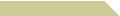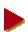HOME COURSES NOTES EXAMS RÉSUMÉ

### TRIAL DIVISION TO TWELVE DIGITS

Enter a positive integer N >>

Reveal the prime factors of N in the textbox below. A question mark (?) appears when a factor is out of range (larger than 10^12), warning that the factorization may be incomplete.

##What is Trial Division?

Trial division is the name of an algorithm which attempts at finding a prime factor of a given positive integer N. The trial is done by repeatedly dividing N by the prime numbers 2, 3, 5, 7, 11, etc., until a factor is found; otherwise N itself is prime.

Note that given a composite N which factors into two or more primes, at most one of the prime factors can be larger than √N while the rest is each bounded by this square root. Therefore, it is not necessary to continue the algorithm once we reach the last prime number p ≤ √N.

##This Implementation

Trial division is very easy to implement in any programming language. In this JavaScript version we store an array of all prime numbers up to 1,000,000. There are exactly 78,498 such primes, the largest of which is p = 999,983. Thus we are set to take on any integer N up to one trillion, i.e., twelve decimal digits, since √1012 = 1,000,000.

Furthermore, once we find a prime factor p, we iterate the trial division with the integer N/p in place of N. In this way, we end up with the complete factorization of N into prime numbers. As already mentioned, if not one factor is found, then the number N proves to be a prime.

JavaScript integers can assume up to 53 bits, where 253 = 9,007,199,254,740,992. In the implementation, we let any N in this range be tested until p = 999,983. However, if the unfactored part remains larger than a trillion by the end of the iterations, then we will be content with an "incomplete" factorization.

Feel free to save or modify a copy of this file for your own use.

##Other Factoring Algorithms

Despite its simple implementation, trial divison is super slow when dealing with large numbers. To tackle a 53-bit integer, for instance, our prime database would swell 70 to 80 times larger than its current size.

For a more advanced and more clever factoring algorithms, try to google the so-called Pollard rho method and p−1 method, or the quadratic sieve method. None of these guarantees a quick result, if successful at all, but with some theoretical help, one can learn the best factoring approach with respect to a given integer range.

There are situations in which one must know simply whether N is prime or composite, without needing the factors of N if composite. This calls for a primality testing algorithm. Generally speaking, testing primes is not as hard as factoring composites, nor as slow. See our testing primes page for an example.